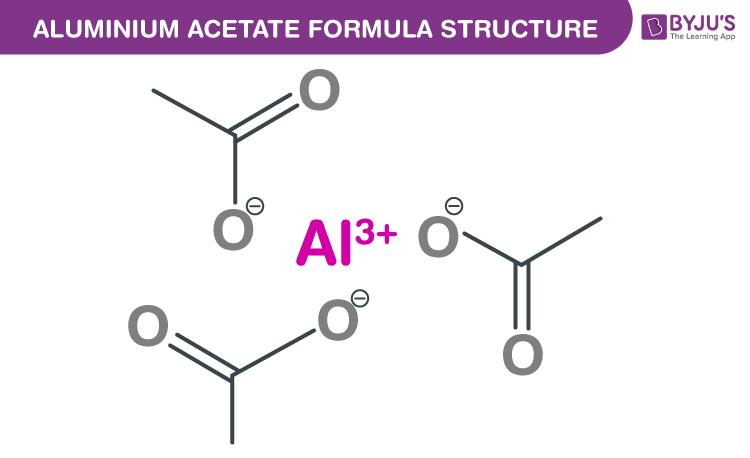# Aluminium Acetate Formula

Aluminium acetate formula, also named as Aluminium ethanoate formula or Aluminum triacetate formula is discussed in this article. The molecular or chemical formula of Aluminium acetate is C6H9AlO6. In the solid form, aluminium acetate exists as three salts under the following these names:

• Neutral aluminium triacetate – Its molecular formula is Al(CH3CO2)3. Formally it is named as aluminium acetate.
• Basic aluminium diacetate – Its molecular formula is HOAl(CH3CO2)2. It is also termed as basic aluminium acetate. Formally it is named as hydroxy aluminium diacetate.
• Basic aluminium monoacetate – Its molecular formula is (HO)2AlCH3CO2. It is also termed as dibasic aluminium acetate. Formally it is named as dihydroxy aluminium acetate.

## Aluminium acetate Formula Structure## Properties Of Aluminium acetate

 Molecular formula C6H9AlO6 Molecular weight 204.114 g/mol Complexity 25.5 Compound canonicalization Yes Covalent bond 4

The number of hydrogen bond donor is equal to zero. The number of hydrogen bond acceptor is equal to six. Monoisotopic mass of Aluminum triacetate is 204.021 g/mol.

### Use Of Ammonium Acetate

• It is widely used as an antiseptic agent.
• It is used to treat inflammation, stinging of the infected skin, itching, and promotes healing.